﻿ Cloud Radiative Feedbacks during the ENSO Cycle Simulated by CAMS-CSM
 J. Meteor. Res.2019, Vol. 33Issue (4): 666-677PDF
http://dx.doi.org/10.1007/s13351-019-8104-3
The Chinese Meteorological Society
0

#### Article Information

CHEN, Lin, Lijuan HUA, Xinyao RONG, et al., 2019.
Cloud Radiative Feedbacks during the ENSO Cycle Simulated by CAMS-CSM. 2019.
J. Meteor. Res., 33(4): 666-677
http://dx.doi.org/10.1007/s13351-019-8104-3

### Article History

in final form April 11, 2019
Cloud Radiative Feedbacks during the ENSO Cycle Simulated by CAMS-CSM
Lin CHEN1,2,3, Lijuan HUA4, Xinyao RONG4, Jian LI4, Lu WANG1, Guo ZHANG4, Ming SUN1, Zi’an GE1
1. Key Laboratory of Meteorological Disaster, Ministry of Education/Joint International Research Laboratory of Climate and Environmental Change/Collaborative Innovation Center on Forecast and Evaluation of Meteorological Disasters, Nanjing University of Information Science & Technology (NUIST), Nanjing 210044;
2. State Key Laboratory of Numerical Modeling for Atmospheric Sciences and Geophysical Fluid Dynamics, Institute of Atmospheric Physics, Chinese Academy of Sciences, Beijing 100029;
3. State Key Laboratory of Loess and Quaternary Geology, Institute of Earth Environment, Chinese Academy of Sciences, Xi’an 710061;
4. State Key Laboratory of Severe Weather, Chinese Academy of Meteorological Sciences, China Meteorological Administration, Beijing 100081
ABSTRACT: This study evaluated the simulated cloud radiative feedbacks (CRF) during the El Niño–Southern Oscillation (ENSO) cycle in the latest version of the Chinese Academy of Meteorological Sciences climate system model (CAMS-CSM). We conducted two experimental model simulations: the Atmospheric Model Intercomparison Project (AMIP), forced by the observed sea surface temperature (SST); and the preindustrial control (PIcontrol), a coupled run without flux correction. We found that both the experiments generally reproduced the observed features of the shortwave and longwave cloud radiative forcing (SWCRF and LWCRF) feedbacks. The AMIP run exhibited better simulation performance in the magnitude and spatial distribution than the PIcontrol run. Furthermore, the simulation biases in SWCRF and LWCRF feedbacks were linked to the biases in the representation of the corresponding total cloud cover and precipitation feedbacks. It is interesting to further find that the simulation bias originating in the atmospheric component was amplified in the PIcontrol run, indicating that the coupling aggravated the simulation bias. Since the PIcontrol run exhibited an apparent mean SST cold bias over the cold tongue, the precipitation response to the SST anomaly (SSTA) changes during the ENSO cycle occurred towards the relatively warmer western equatorial Pacific. Thus, the corresponding cloud cover and CRF shifted westward and showed a weaker magnitude in the PIcontrol run versus observational data. In contrast, the AMIP run was forced by the observational SST, hence representing a more realistic CRF. Our results demonstrate the challenges of simulating CRF in coupled models. This study also underscores the necessity of realistically representing the climatological mean state when simulating CRF during the ENSO cycle.
Key words: ENSO     CAMS climate system model     cloud radiative feedbacks     ENSO cycle
1 Introduction

Clouds could regulate the global energy distribution via radiative effects and thereby altering the atmospheric circulation, which in turn modulates the clouds and their effects (Houghton et al., 2001; Stephens, 2005). A slight change in the cloud radiative effects can greatly affect the climate sensitivity to anthropogenic effects (Houghton et al., 2001). However, cloud radiative feedbacks (CRF) simulated by current climate models have been faced with challenges for decades and are recognized as a primary source of uncertainty in the climate simulation and prediction (Li, 1997; Bony and Dufresne, 2005; Soden and Held, 2006; Sun et al., 2006, 2009; Lin, 2007; Randall et al., 2007; Guilyardi et al., 2009; Lloyd et al., 2009; Su et al., 2010; Li et al., 2014, 2015; Zheng et al., 2014; Fang et al., 2015; Pu et al., 2016; Tang et al., 2016).

While many studies have been devoted to improving cloud radiative simulations (e.g., Sun et al., 2003, 2006, 2009; Lloyd et al., 2009, 2011, 2012; Chen et al., 2013, 2018a, b; Chen and Yu, 2014; Li et al., 2014, 2015; Zheng et al., 2014; Fang et al., 2015), refining that these simulations is still a key challenge. Previous studies have determined a common bias in CRF simulations: the simulated response of shortwave and longwave cloud radiative forcing (SWCRF and LWCRF) feedbacks to SSTA changes in the tropical Pacific region is underestimated in the phase 3 of Coupled Model Intercomparison Project (CMIP3) models (Sun et al., 2006, 2009). The common biases of CRF exhibited in CMIP3 models still remain prevalent in the phase 5 of Coupled Model Intercomparison Project (CMIP5) models (Chen et al., 2013; Li et al., 2014, 2015). The underestimation of CRF reduces the utility of such models in climate predictions, as reported by the IPCC (Randall et al., 2007). Thus, continuous attention needs to be paid to improve CRF simulations.

In general, two methodologies have been applied to evaluate cloud feedbacks in model simulations. The first method examines the response of clouds to a prescribed global mean surface temperature or model-projected global mean surface temperature changes (e.g., Cess et al., 1990; Soden and Vecchi, 2011). These studies pointed out that there is a large spread in the simulated cloud feedbacks (especially the shortwave-related cloud radiative effects) among model simulations. In this method, cloud radiative forcing is usually regressed against the global mean surface temperature changes, which means that the spatial pattern of sea surface temperature (SST) change is neglected. Since it is unlikely that SST will increase uniformly in space under the anthropogenic climate change (Xie et al., 2010), this method has its limitation. To avoid this issue, the second method, which has been widely applied in numerous studies, investigates the cloud radiative forcing in response to SST changes on El Niño–Southern Oscillation (ENSO) timescales (e.g.,Zhang and Sun, 2006, 2008; Lloyd et al., 2009; Wu et al., 2011; Chen et al., 2013, 2016a; Li et al., 2014, 2015). Comparing the response of cloud radiative forcing to ENSO SST changes in the observational data versus model simulations allows one to quantify the ability of a climate model to simulate CRF. We apply this methodology in the present study.

Recently, the Chinese Academy of Meteorological Sciences (CAMS) developed a new climate system model (CAMS-CSM). Based on a large amount of experiments, we recently set the latest version of CAMS-CSM, which was intended to participate in the incoming CMIP6. Motivated by this, we aim to examine the performance of CRF simulations in the latest version of CAMS-CSM and present possible explanations for the simulation bias in the current model.

In the remaining of this paper, Section 2 provides the introduction of the model and datasets used in this study. The specific performance of CRF simulated by the CAMS-CSM is provided in Section 3. The possible explanation for the simulation bias in the CAMS-CSM is analyzed in Section 4. Finally, the conclusions and discussion are given in the end.

2 Methods 2.1 CAMS-CMS

CAMS-CSM comprises four discrete units: atmospheric, oceanic, sea ice, and land. For the atmospheric component, CAMS-CSM uses ECHAM5 (v5.4; Roeckner et al., 2003), a modified atmospheric general circulation model (AGCM) developed at the Max Planck Institute for Meteorology (MPI-Met). The oceanic component is represented by the Modular Ocean Model (MOM4; Griffies et al., 2004), which was developed at the Geophysical Fluid Dynamics Laboratory (GFDL). The sea–ice component relies on the GFDL Sea Ice Simulator (SIS; Winton, 2000), and the land component uses the Common Land Model (CoLM; Dai et al., 2003). For flux/state calculations and interpolations between component models, CAMS-CSM utilized the GFDL Flexible Modelling System (FMS) coupler. CAMS-CSM includes refinements based on Hua et al. (2018), and a detailed description was introduced in Rong et al. (2018). CAMS-CSM has been applied in numerous studies (Hua and Chen, 2019; Hua et al., 2019; Wang, et al., 2019). Outputs from the Atmospheric Model Intercomparison Project (AMIP) and preindustrial control (PIcontrol) runs are used for analysis in this study. The AMIP run ( https://cmip.llnl.gov/cmip5/experiment_design.html) was an AGCM run forced by the observed SSTs and sea ice prescribed for 1979–2013. This means that in AMIP simulations, SSTs are specified based on observations. In the present study, in order to match the specific period of the observed datasets [since majority of the variables, such as the shortwave heat flux, total cloud cover, liquid water path (LWP) etc. analyzed in this study were derived from the International Satellite Cloud Climatology Project (ISCCP; 1983–2009)], we utilized the AMIP outputs during 1984–2009. On the other hand, the PIcontrol run is a Coupled General Circulation Model (CGCM) control run without any flux correction. In the PIcontrol experiment, the model was firstly integrated for more than thousands of years, and after the modeling climate reached the quasi-equilibrium state (Rong et al., 2018), we utilized the last 50-yr outputs (model years 2525–74) from the PIcontrol run for analysis. For more details about the AMIP and PIcontrol experiments, readers can refer to Rong et al. (2018). Plenty of previous studies have employed the PIcontrol and AMIP runs to investigate the mechanism on ENSO-related radiative feedbacks issues (e.g., Lloyd et al., 2011; Chen et al., 2013; Li et al., 2015; Ferrett and Collins, 2016; Ferrett et al., 2018) through comparing the PIcontrol simulation results with those of AMIP. For instance, Ferrett et al. (2018) examined the difference in radiative feedbacks with reference to the differing mean states between CMIP and AMIP; Li et al. (2015) also discussed the cold tongue bias with respect to CMIP–AMIP feedback differences.

2.2 Data

Observational and reanalysis datasets used in this study include the following: (1) SST from the Hadley Center Sea Ice and Sea Surface Temperature dataset (HadISST1; Rayner et al., 2003) during 1984–2009; (2) monthly radiative fluxes, total cloud amount, and LWP from the ISCCP dataset (Zhang et al., 2004) during 1984–2009; (3) monthly precipitation from the Global Precipitation Climatology Project (GPCP; Huffman et al., 2009) during 1984–2009; and (4) monthly vertical velocity from the ERA-Interim (Dee et al., 2011) during 1984–2009.

3 Performance of CRF in the CAMS-CSM

SWCRF at the top of the atmosphere (TOA) was defined as:

 ${\rm{SWCRF = SW - S}}{{\rm{W}}_{\rm c}},$ (1)

where SW and SWc represent the TOA net downward solar radiation for average cloudy conditions and clear sky, respectively. Following previous studies (Sun et al., 2006, 2009; Chen et al., 2013; Li et al., 2014, 2015), we obtained the SWCRF response to SSTA changes during the ENSO cycle by regressing the anomalous SWCRF onto SSTA averaged in the central-eastern equatorial Pacific (CEEP) region (5°S–5°N, 150°E–110°W). It is noted that all the anomaly fields throughout this study are defined as the deviation relative to their respective climatological seasonal cycle.

The results presented in Fig. 1 illustrate a basin-wide view of the response of SWCRF to SSTA changes during the ENSO cycle (hereafter, “SWCRF feedback”) for the observational data, AMIP run, and PIcontrol run. In the observation, the pronounced negative SWCRF anomalies were noted over the central equatorial Pacific (CEP; Fig. 1a). The SWCRF feedback exhibited an anomalous negative center to the west of dateline. As shown in Fig. 1b, the negative SWCRF feedback in CEP was simulated well in the AMIP run, although the magnitude was weaker than the observation. However, the SWCRF feedback simulation bias was amplified in the PIcontrol run. Specifically, the magnitude of the negative SWCRF feedback in CEP was underestimated and the negative center shifted westward in the PIcontrol run relative to both the AMIP run and observation. Furthermore, we calculated the spatial pattern correlation of the SWCRF feedback over the tropical Pacific region (30°S–30°N, 110°E–70°W) between the simulations and observation. The results show that the spatial correlation coefficient was larger between the AMIP run and observational result (0.81) than that between the PIcontrol run and observational result (0.59), which confirms that the SWCRF feedback bias originating in the AMIP run was amplified in the PIcontrol run after the air–sea coupling.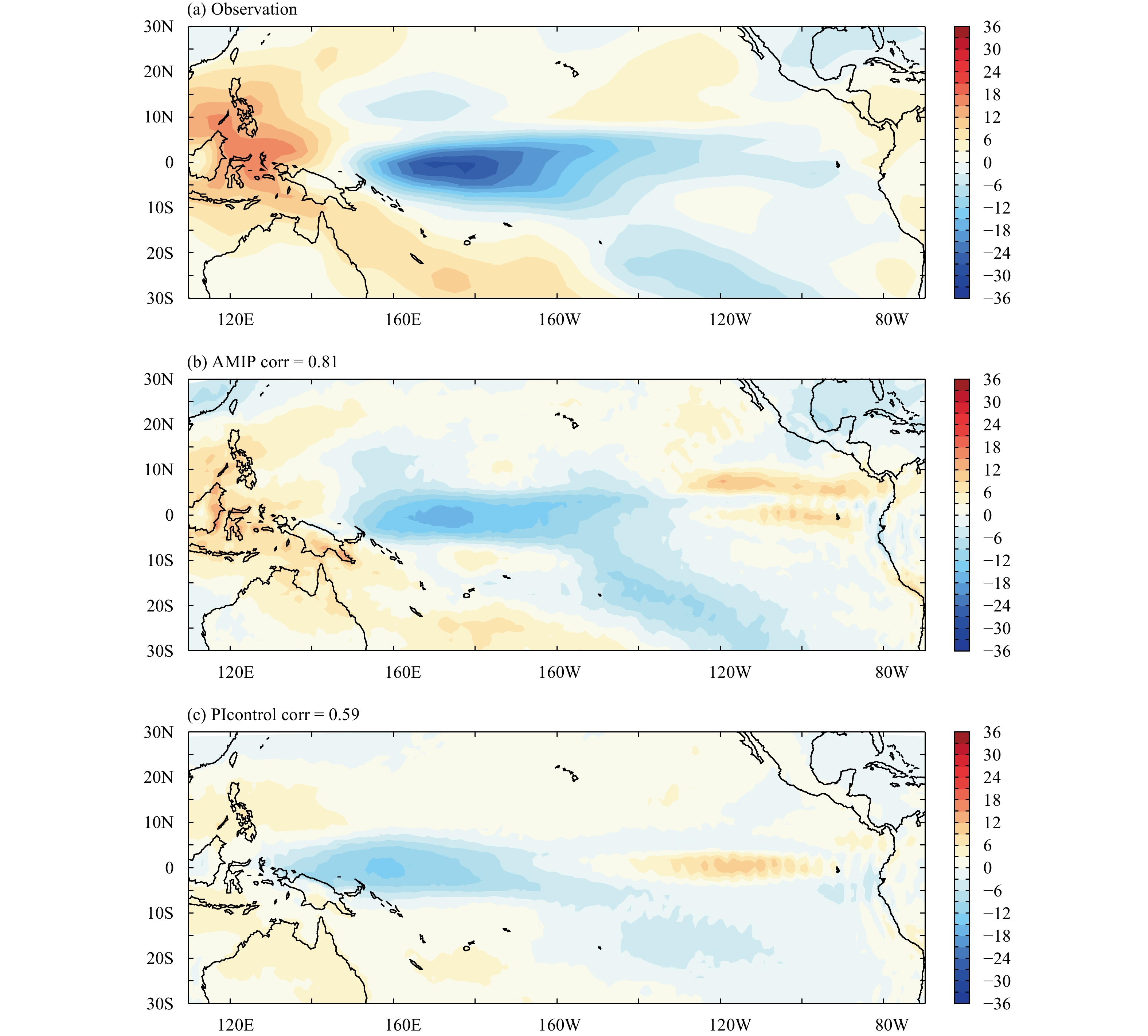Figure 1 The response of SWCRF to SSTA changes (W m−2 K−1) during the ENSO cycle for the (a) observation, (b) AMIP run, and (c) PIcontrol run. Spatial pattern correlation coefficients between the two simulations and observational data are shown in Figs. 1b, c.

LWCRF was defined as:

 ${\rm{LWCRF}} = {\rm{ }}{F_{\rm c}}-F,$ (2)

where F and Fc indicate the outgoing longwave radiation for average cloudy conditions and clear sky, respectively.

Figure 2 displays the horizontal pattern of the LWCRF response to SSTA changes during the ENSO cycle (hereafter “LWCRF feedback”) in the observation, AMIP and PIcontrol runs. In the observational data, the pronounced positive LWCRF anomalies were located over CEP (Fig. 2a). The LWCRF feedback had an anomalous positive center near the dateline. As shown in Fig. 2b, the positive LWCRF feedback in CEP was simulated well in the AMIP run, although the magnitude was slightly weaker than the observation. As in the SWCRF feedback, simulation bias in the LWCRF feedback was aggravated in the PIcontrol run. The positive LWCRF feedback shifted westward in the PIcontrol run relative to the AMIP run and observation, and the magnitude of the negative SWCRF feedback in CEP was underestimated in the PIcontrol run. We calculated the spatial pattern correlation of the LWCRF feedback over the tropical Pacific (30°S–30°N, 110°E–70°W) between the simulations and observation. Like the SWCRF feedback, the AMIP run had a higher pattern correlation coefficient with the observation (0.93) than the PIcontrol run (0.79). This also indicated that the simulation bias of LWCRF feedback originating in the AMIP run was amplified in the coupled run.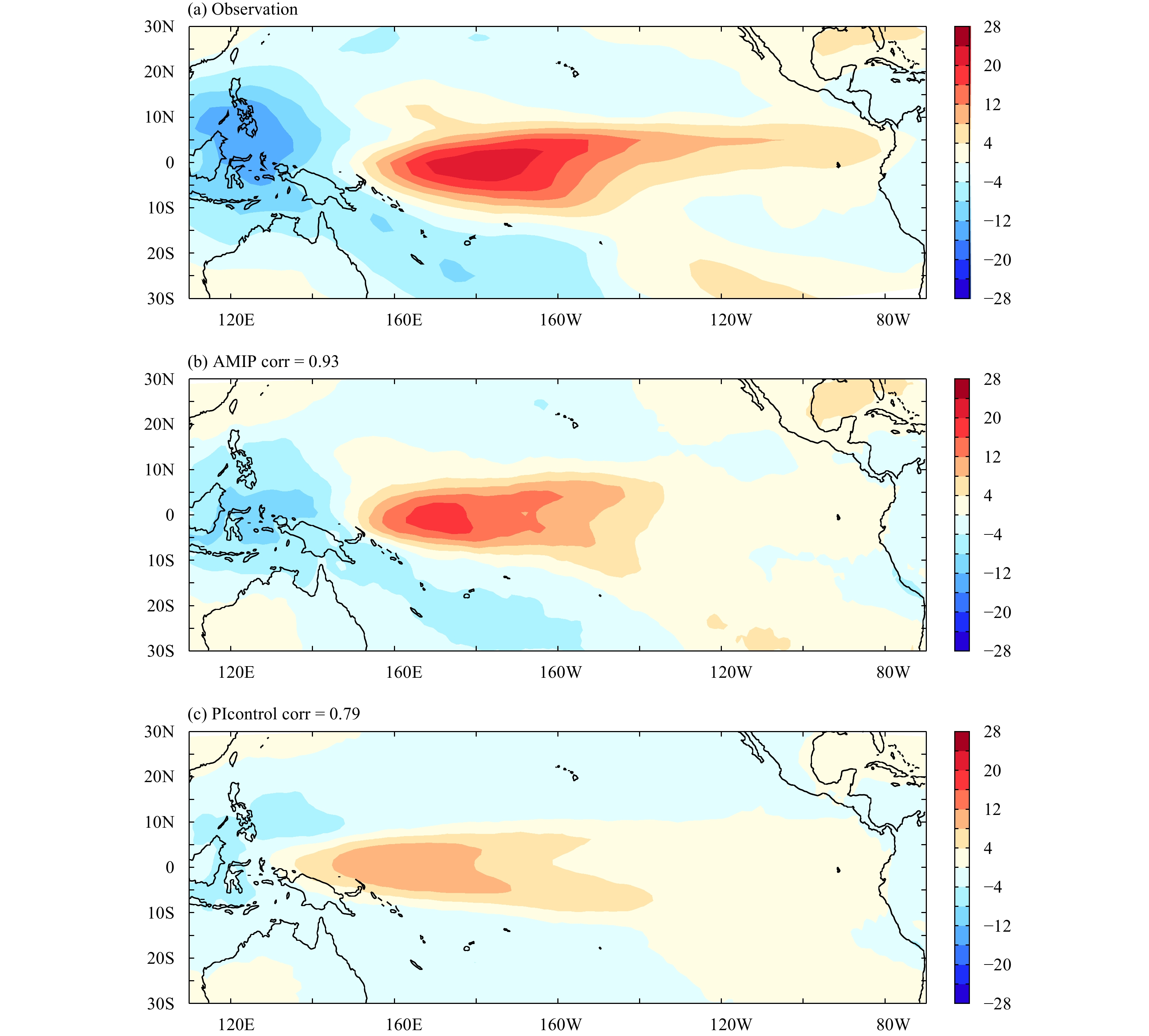Figure 2 As in Fig. 1, but for the response of LWCRF to SSTA changes (W m−2 K−1) during the ENSO cycle.

In summary, both the AMIP and PIcontrol runs from the CAMS-CSM generally captured the characteristics of CRF during the ENSO cycle. Specifically, the AMIP run yielded better agreements with the observational data than the PIcontrol run in terms of both the SWCRF and LWCRF feedbacks. CRF (including both SWCRF and LWCRF) represented by the coupled run were considerably underestimated, and exhibited a westward shift compared to the observational data. It is worth noting that, besides the coupled model analyzed in this study, the simulated CRF biases are also prevalent in the current coupled models (e.g., Chen et al., 2013).

4 Possible explanations for the cloud radiative simulation bias in CAMS-CSM

Based on the assessment above, we endeavored to understand the physical components of the cloud radiative simulation bias in the CAMS-CSM, particularly in the coupled version (PIcontrol run).

Following the surface shortwave heat flux feedback decomposition method proposed by some recent studies (Li et al., 2014, 2015; Ferrett et al., 2018), the response of the shortwave heat flux at surface (SWsfc) to SSTA (i.e., ${\alpha _{{\rm{SW}}}}$ ), which is calculated by the linear regression of downward SWsfc seasonal anomalies averaged over the Niño-3 region (5°S–5°N, 150°–90°W) on positive Niño 3 SSTAs, may be decomposed into the following components:

 $\begin{split} {\alpha _{{\rm{SW}}}} = & \; \dfrac{{\partial {\rm{SW}}}}{{\partial {\rm{CLD}}}}\dfrac{{d{\rm{CLD}}}}{{d{\rm{SST}}}} + \dfrac{{\partial {\rm{SW}}}}{{\partial {\rm{LWP}}}}\dfrac{{d{\rm{LWP}}}}{{d{\rm{SST}}}}\\ \;\;\;\; = & \; \dfrac{{\partial {\rm{SW}}}}{{\partial {\rm{CLD}}}}\left(\dfrac{{\partial {\rm{CLD}}}}{{\partial \omega {\rm{500}}}}\dfrac{{d\omega {\rm{500}}}}{{d{\rm{SST}}}} + \dfrac{{\partial {\rm{CLD}}}}{{\partial {\rm{RH}}}}\dfrac{{d{\rm{RH}}}}{{d{\rm{SST}}}}\right) + \dfrac{{\partial {\rm{SW}}}}{{\partial {\rm{LWP}}}}\dfrac{{d{\rm{LWP}}}}{{d{\rm{SST}}}}\\ \;\;\;\;{\rm{= }} & \; \dfrac{{\partial {\rm{SW}}}}{{\partial {\rm{CLD}}}}\dfrac{{\partial {\rm{CLD}}}}{{\partial \omega {\rm{500}}}}\dfrac{{d\omega {\rm{500}}}}{{d{\rm{SST}}}}{\rm{ + }}\dfrac{{\partial {\rm{SW}}}}{{\partial {\rm{CLD}}}}\dfrac{{\partial {\rm{CLD}}}}{{\partial {\rm{RH}}}}\dfrac{{d{\rm{RH}}}}{{d{\rm{SST}}}} + \dfrac{{\partial {\rm{SW}}}}{{\partial {\rm{LWP}}}}\dfrac{{d{\rm{LWP}}}}{{d{\rm{SST}}}}\\ {\rm{= }} & \;\;{\rm{DYF}}{\rm{ + }}{\rm{RHF}}{\rm{ + }}{\rm{LWPF},} \end{split}$ (3)

where ${{\partial {\rm{SW}}} / {\partial {\rm{CLD}}}}$ denotes the response of the SWsfc anomaly to total cloud cover (CLD) anomaly; dCLD/dSST denotes the response of the CLD anomaly to SSTA; ${{\partial {\rm{SW}}} / {\partial {\rm{LWP}}}}$ is the response of the SWsfc anomaly to LWP anomaly; dLWP/dSST is the response of the LWP anomaly to SSTA; ${{\partial {\rm{CLD}}} / {\partial \omega {\rm{500}}}}$ is the response of the CLD anomaly to anomalous vertical velocity at 500 hPa ( $\omega {\rm{500}}$ ); ${{{{d}}\omega {\rm{500}}} / {{d \rm{SST}}}}$ is the response of the $\omega {\rm{500}}$ anomaly to SSTA; ${{\partial {\rm{CLD}}} / {\partial {\rm{RH}}}}$ is the response of the CLD anomaly to surface relative humidity (RH) anomaly; and dRH/dSST is the response of the RH anomaly to SSTA. In this way, the SWsfc feedback can be estimated by the sum of the dynamical cloud feedback (DYF), surface RH cloud feedback (RHF), and LWP feedback (LWPF).

By using the decomposition method above, we calculated the ${\alpha _{{\rm{SW}}}}$ , estimated DYF, RHF, LWPF from the renalaysis (green bars), AMIP run (blue), and PIcontrol run (red). Note that following Ferrett et al. (2018), we here computed these feedbacks by using only positive Niño-3 SSTAs (SSTA > 0 K), and we utilized the datasets covering 1984−2009, including (1) ISCCP, which provides the SW heat flux at the surface, CLD, and vertically integrated LWP; and (2) ERA-Interim, which provides the vertical velocity at 500 hPa and surface RH.

As shown in Fig. 3, ${\alpha _{{\rm{SW}}}}$ over the Niño 3 region from the observation, AMIP and PIcontrol runs are respectively −10.7, −9.2, and −5.4 W m −2. According to the aforementioned idea in decomposing the processes associated with ${\alpha _{{\rm{SW}}}}$ , we further found that the biases in simulating ${\alpha _{{\rm{SW}}}}$ in both AMIP and PIcontrol runs are primarily attributed to the bias in DYF, while the biases in simulating RHF and LWPF (which are associated with the atmospheric processes, such as RH and LWP) are relatively small. Therefore, next we will mainly focus on the bias in simulating the dynamical processes, such as the response of the anomalous CLD and $\omega {\rm{500}}$ to SSTA.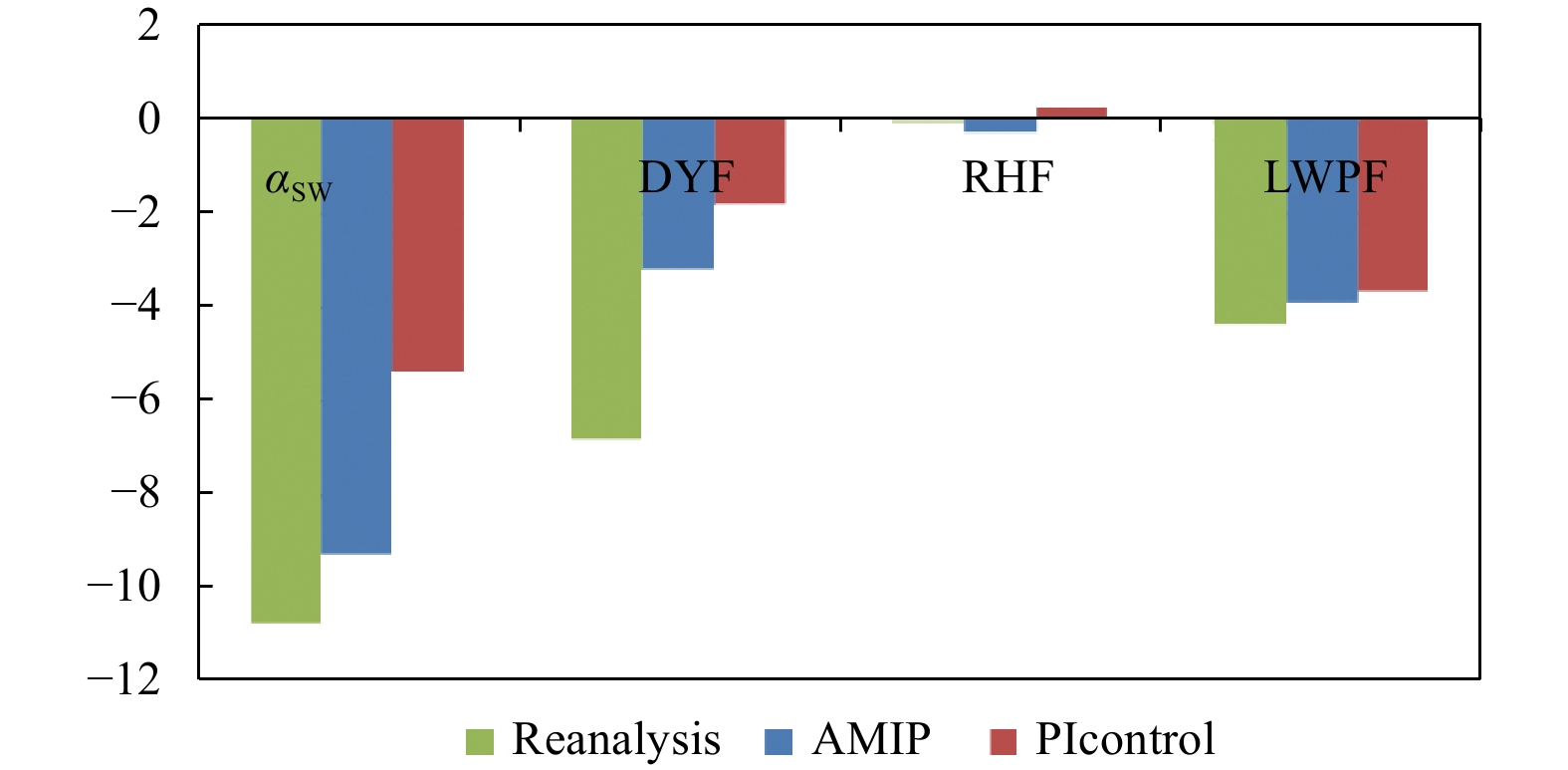Figure 3 From left to right, bars show the SWsfc feedback ( ${\alpha _{{\rm{SW}}}}$ ; m−2 K−1), DYF, RHF, and LWPF from the reanalyses (green bars), AMIP run (blue), and PIcontrol run (red). Note that the feedbacks shown here were calculated by using only the positive Niño 3 SSTAs (SSTA > 0 K).

Actually, previous studies have suggested that SWCRF and LWCRF feedbacks are linked with CLD and precipitation feedbacks (Sun et al., 2009; Chen et al., 2013; Li et al., 2014, 2015; Ferrett et al., 2018). Thus, we first examined the response of CLD to SSTA changes during the ENSO cycle (hereafter “CLD feedback”) in the observational data, AMIP and PIcontrol runs (Figs. 4ac). The overall performance in the CLD feedback simulation was consistent with that in the simulation of CRF. Again, it was noted that a relatively small simulation bias originating in the atmospheric component (AMIP run) was amplified in the coupled run (PIcontrol run). For example, the pattern correlation coefficients of the observed CLD feedback and two simulations (AMIP and PIcontrol runs) were 0.84 and 0.72, respectively. In addition to the spatial pattern of CLD feedback, we also provided the vertical profile of cloud fraction response to SSTA changes during the ENSO cycle for AMIP and PIcontrol runs (Fig. 5). From the view of vertical profile, there is no obvious difference in the vertical distribution of cloud fraction response between two simulations. The main difference between the AMIP and PIcontrol runs lies in the latitudinal position of the cloud fraction response (e.g., boundary of the positive and negative cloud fraction response locates at 150°E in the AMIP run, but 130°E in the PIcontrol run), which is consistent with the spatial pattern of CLD feedback. Then, we further examined the corresponding response of precipitation to SSTA changes during the ENSO cycle (hereafter “PR feedback”) in the observation and two simulations (Fig. 6). It was found that the PR feedback showed a similar manner in the simulation bias, which was more severe in the PIcontrol run than AMIP, as indicated by the contrasting pattern correlation coefficients (0.72 vs. 0.92).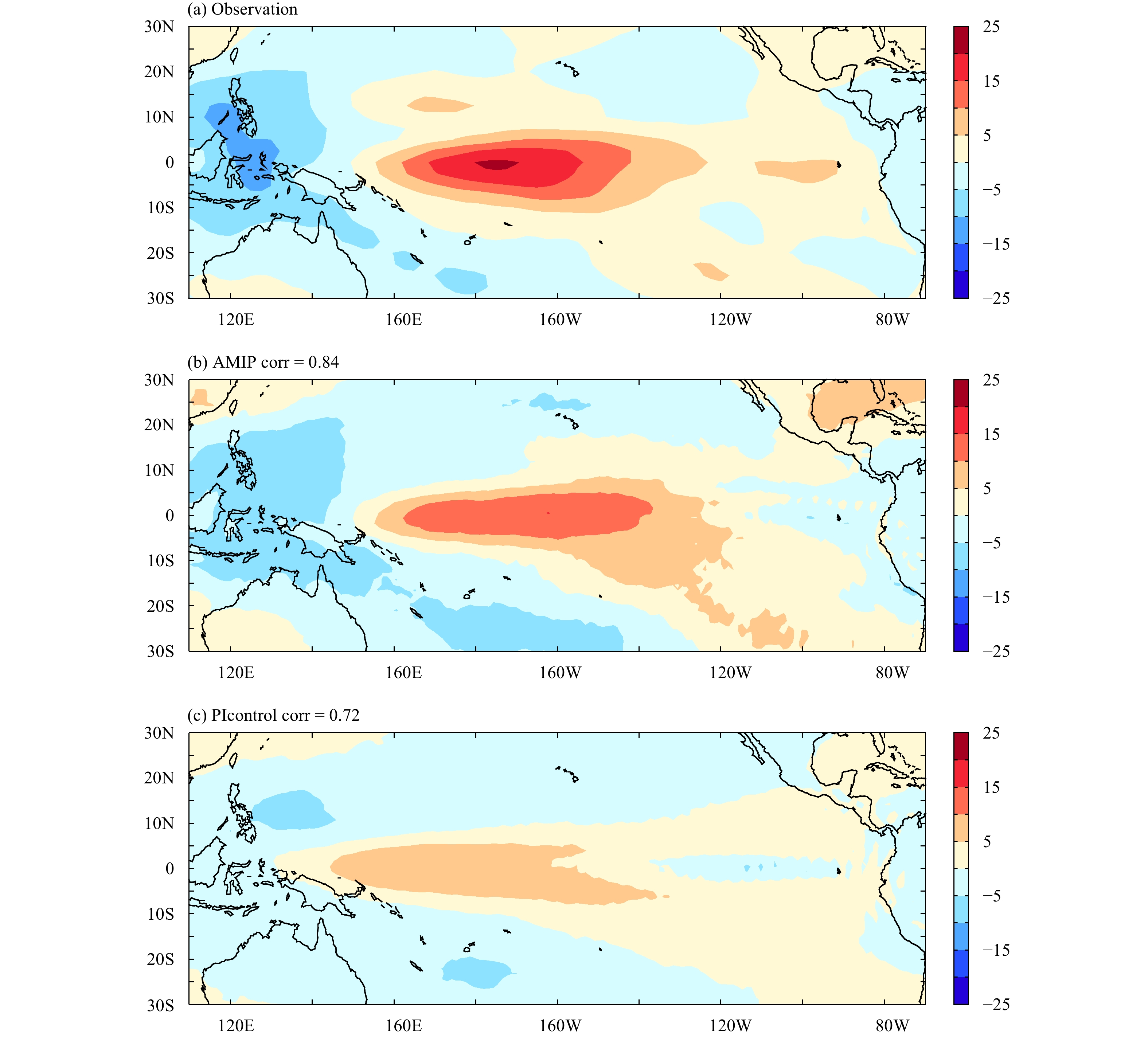Figure 4 As in Fig. 1, but for the response of CLD to SSTA changes (% K−1) during the ENSO cycle.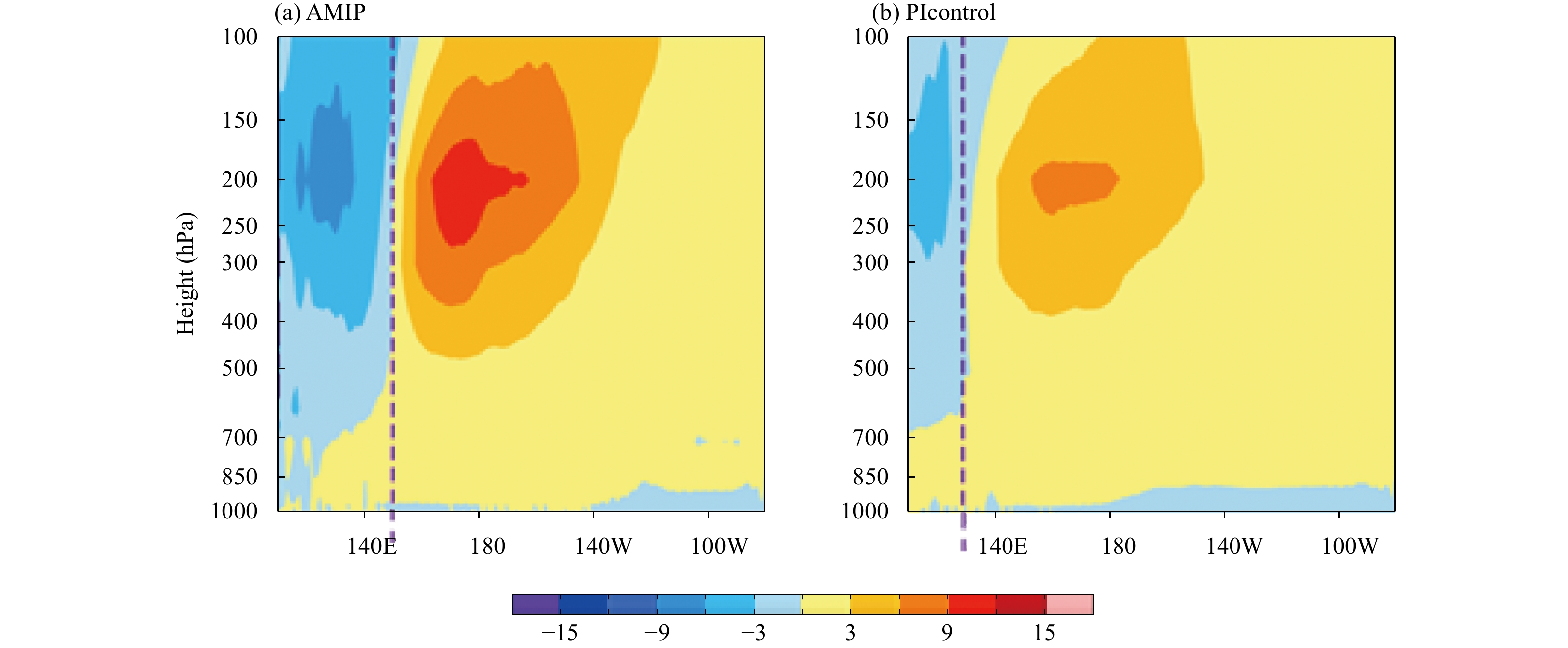Figure 5 The cloud fraction response to SSTA changes (% K−1) during the ENSO cycle along the equator (averaged between 5°S and 5°N) for (a) AMIP and (b) PIcontrol runs.Figure 6 As in Fig. 1, but for the response of precipitation to SSTA changes (mm day−1 K−1) during the ENSO cycle.

The simulation biases in representing both the CLD and PR feedback indicate that the main bias lies in the maximum centers of feedbacks. For example, the maxi-mum centers for response of precipitation and the corresponding CLD to SSTA changes during the ENSO cycle were represented correctly in the AMIP run, but the maximum centers of the CLD and PR feedback in the PIcontrol run show a westward shift, analogously. The westward shift bias also presented in response of the convection to SSTA changes during the ENSO cycle. As shown in Fig. 7, the atmospheric vertical velocity response to SSTA changes during the ENSO cycle exhibited the same westward shift bias in the PIcontrol run. Positions of the anomalous ascending motion in response to SSTA changes during the ENSO cycle in the AMIP run generally agreed with the observation, whereas anomalous ascending motion in the PIcontrol run exhibited a westward shift relative to the AMIP run and observation.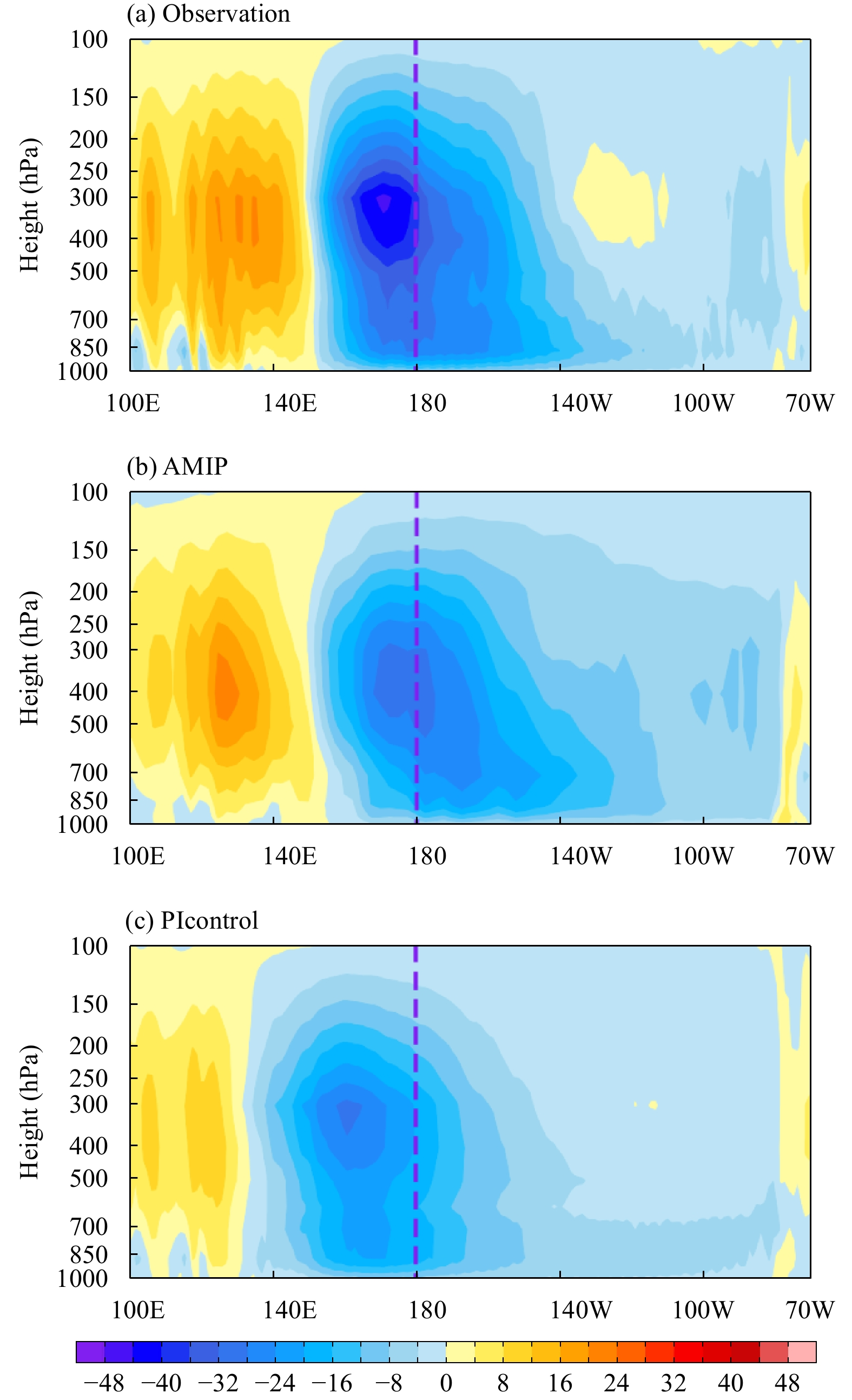Figure 7 The vertical velocity response to SSTA changes (hPa day−1 K−1) during the ENSO cycle along the equator (averaged between 5°S and 5°N) for the (a) observation, (b) AMIP run, and (c) PIcontrol run.

One may be wondering that why the CLD feedback in the AMIP run is underestimated but response of the precipitation and atmospheric vertical velocity at 500 hPa to SSTA changes during the ENSO cycle is overestimated in the AMIP run. As suggested by some recent studies (Li et al., 2014; Ferrett et al., 2018), the bias in the CLD feedback is not purely dependent on the dynamical processes’ feedbacks, such as response of the precipitation and dynamical vertical velocity to SSTA. Rather, the bias in the CLD feedback may be also related to some other biases in AGCM, such as response of the CLD anomaly to surface RH anomaly and response of the RH anomaly to SSTA. More in-depth study about the bias in the magnitude of CLD feedback in the atmosphere-only GCM needs to be further investigated in the future.

A westward shift bias in simulating PR and CLD feedbacks directly leads to a westward shift when simulating CRF, as in SWCRF and LWCRF feedbacks. This westward shift bias would also influence the ENSO evolution and amplitude. For example, the SWCRF feedback is a dominant thermodynamic damping feedback during an ENSO cycle and would suppress the ENSO development (Li et al., 2014, 2015; Chen L. et al., 2016, 2017; Chen M. C. et al., 2016). The CEEP response to SSTA changes during the ENSO cycle (the westward shift bias in the PIcontrol run) would lead to a nearly zero or slightly positive SWCRF feedback in the eastern equatorial Pacific (EEP). This underestimation of the SWCRF feedback would decrease the ocean–atmosphere stability, and the simulated ENSO amplitude would tend to be larger than the observed (Kim and Jin, 2011a, b; Kim et al., 2014).

What caused the westward shift bias in PR, CLD, and corresponding CRF in the PIcontrol run compared to the observational data? The leading factor contributing to the bias was the excessive cold tongue (the cold bias in the mean SST) in the PIcontrol run, since there is no obvious difference in terms of the spatial pattern of ENSO-related SSTA between AMIP and PIcontrol runs (figure omitted). As shown by data in Fig. 8, the cold tongue simulated by the PIcontrol run is colder than the observational data. The cold SST bias prevents the total SST (mean SST plus SSTA) from exceeding the convection threshold when a perturbed warm SST anomaly (SSTA) of El Niño occurs in CEEP. Thus, the convection ultimately shifts toward the relatively warmer western equatorial Pacific region. Recalling that the AMIP run was forced by the observed SST (SST in the AMIP run was realistic without any cold bias), the coupled (PIcontrol) version of CAMS-CSM exhibited relatively larger biases than the AMIP run when simulating the spatial pattern and magnitude of the precipitation feedback and associated CRF during the ENSO cycle.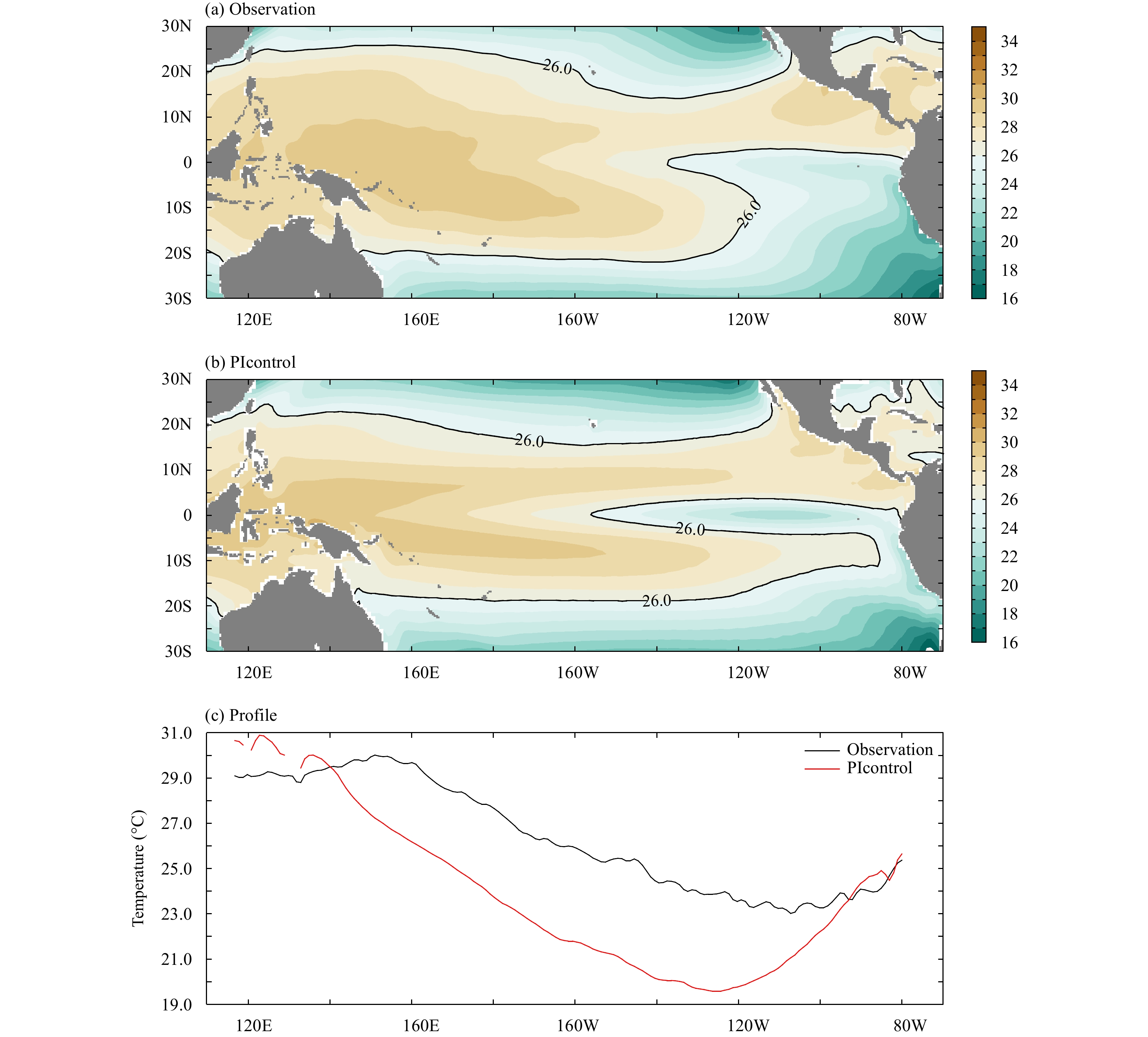Figure 8 Climatological SST (°C) for the (a) observational data and (b) PIcontrol run. (c) The climatological SST profile along the equator (averaged between 5°S and 5°N), and the y-axis denotes the unit of temperature (°C).

It is worth noting that as the atmospheric component of CAMS-CSM is not perfect, the AMIP run itself shows some bias in simulating CRF. The deficiency in the AMIP run largely arises from model system biases in the atmospheric component, such as unreasonable parameterization schemes (e.g., Li et al., 2014, 2015). But the main point that we underscore here by analyzing the two simulations from CAMS-CSM, is that the reasonable simulation of mean SST is essential for the reasonable simulation of CRF on interannual timescales; otherwise, the cold SST bias causes a relatively small simulation bias originating in the atmospheric component to be easily amplified in the coupled run.

5 Conclusions and Discussion

The major modulation of clouds in the climate system and uncertainties in cloud feedbacks necessitate the careful evaluation of CRF in response to SSTA changes during the ENSO cycle. Here, we evaluated the simulation of CRF and other relevant feedbacks from AMIP and PIcontrol runs in the newest coupled model developed by CAMS (CAMS-CSM). Main findings of this study are summarized in the following statements.

(1) Overall, the simulated SWCRF and LWCRF feedbacks can reproduce the observed spatial pattern derived from both the AMIP and PIcontrol runs. The AMIP run exhibited the better simulation of CRF than PIcontrol. The relatively small bias originating from the AMIP run tended to be easily amplified in the corresponding PIcontrol run. Specifically, the SWCRF feedback in the PIcontrol run exhibited a westward shift bias when compared to the observation and AMIP run, which is underestimated in EEP (since the overall spatial patterns, especially the maximum feedback centers, shift westward).

(2) We firstly applied the ${\alpha _{{\rm{SW}}}}$ decomposition method to diagnose the processes associated with ${\alpha _{{\rm{SW}}}}$ , and found that the biases in simulating ${\alpha _{{\rm{SW}}}}$ from both AMIP and PIcontrol runs are primarily attributed to the bias in DYF, while the biases in simulating RHF and LWPF are relatively small. Therefore, we further investigated the bias in simulating the dynamical processes. It is found that the biases in SWCRF and LWCRF feedbacks can be traced back to biases in the corresponding CLD and PR feedbacks. CLD and PR feedbacks also exhibited the analogous biases, including the westward shift distribution and underestimated magnitude in EEP. Again, the biases in simulating CLD and PR feedbacks in the coupled run were more pronounced than those from AMIP run. CLD and PR feedbacks in the coupled run showed an apparent westward shift compared to the AMIP run and observation, hence appearing to be underestimated in the eastern Pacific.

(3) We found that the simulation biases in CLD and PR feedbacks for the PIcontrol run were mainly due to the cold SST bias over CEEP. Because SST in CEEP in the PIcontrol run was colder than the observational data, when a perturbed positive SSTA with a certain magnitude appeared in CEEP, the total SST could not exceed the threshold in the PIcontrol run, and hence PR and CLD feedbacks occurred towards the relatively warmer western-CEP. Thus, the corresponding CRF in the coupled run shifted westward and exhibited a weaker magnitude in EEP.

It is worth noting that the better improvement of radiative feedbacks in the AMIP run than that in the PIcontrol run may be model-dependent, since this study is about the single model. Additionally, although the cold SST bias over CEEP in the coupled run is an important factor in cloud radiative feedback biases, other factors also contribute to inaccurate cloud radiative feedback simulations in CAMS-CSM (as evidenced by the relatively small bias in the AMIP run, despite integration of the observed SST). Other factors may include model system biases in the convection parameterization schemes, cloud microphysical schemes, and model resolution (Kim et al., 2008; Li and Zhang, 2008; Toniazzo et al., 2008; Li et al., 2014, 2015). Specifically, recent studies (Li et al., 2014, 2015) indicated that the SWCRF feedback simulation during an ENSO cycle was improved by tuning 14 uncertain parameters associated with the deep convection scheme, convective cloud fraction, and cloud microphysical scheme. In addition to the updated parameterization schemes, these studies also demonstrated that the coordination among physical schemes is the key to improving simulations in ENSO feedbacks. While further study is necessary, we identified that minimizing errors in the atmospheric component of the CAMS coupled model is necessary to prevent the bias amplification and subsequent underestimation of SWCRF and LWCRF feedbacks.

References